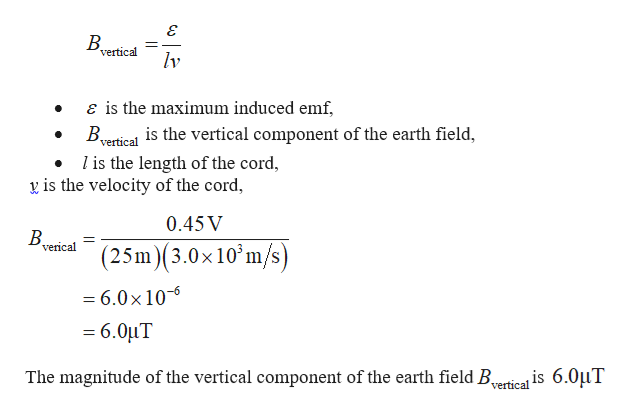# An astronaut is connected to her spacecraft by a25-m-long tether cord as she and the spacecraft orbit Earth ina circular path at a speed of 3.0 x 103 m/s. At one instant, thevoltage measured between the ends of a wire embedded in thecord is measured to be 0.45 V. Assume the long dimension ofthe cord is perpendicular to the vertical component of Earth’smagnetic field at that instant. (a) What is the magnitude of thevertical component of Earth’s field at this location? (b) Doesthe measured voltage change as the system moves from onelocation to another? Explain.

Question
1 views

An astronaut is connected to her spacecraft by a
25-m-long tether cord as she and the spacecraft orbit Earth in
a circular path at a speed of 3.0 x 103 m/s. At one instant, the
voltage measured between the ends of a wire embedded in the
cord is measured to be 0.45 V. Assume the long dimension of
the cord is perpendicular to the vertical component of Earth’s
magnetic field at that instant. (a) What is the magnitude of the
vertical component of Earth’s field at this location? (b) Does
the measured voltage change as the system moves from one
location to another? Explain.

check_circle

Step 1

Formula to calculate magnitude of the vertic...help_outlineImage TranscriptioncloseB. vertical lv ɛ is the maximum induced emf, Byertical is the vertical component of the earth field, l is the length of the cord, y is the velocity of the cord, 0.45 V B. verical (25m)(3.0x10°m/s) = 6.0×106 = 6.0µT The magnitude of the vertical component of the earth field Bertica is 6.0µT fullscreen

### Want to see the full answer?

See Solution

#### Want to see this answer and more?

Solutions are written by subject experts who are available 24/7. Questions are typically answered within 1 hour.*

See Solution
*Response times may vary by subject and question.
Tagged in

### Physics